# 林轩田机器学习基石课程学习笔记10 — Logistic Regression

## 一、Logistic Regression Problem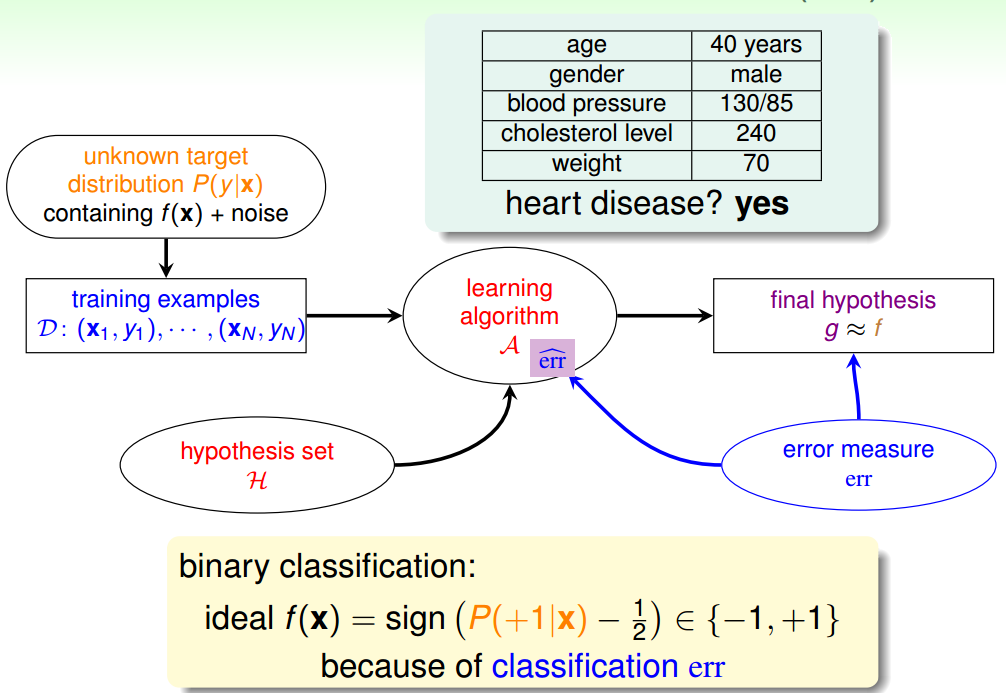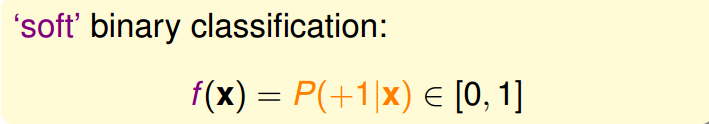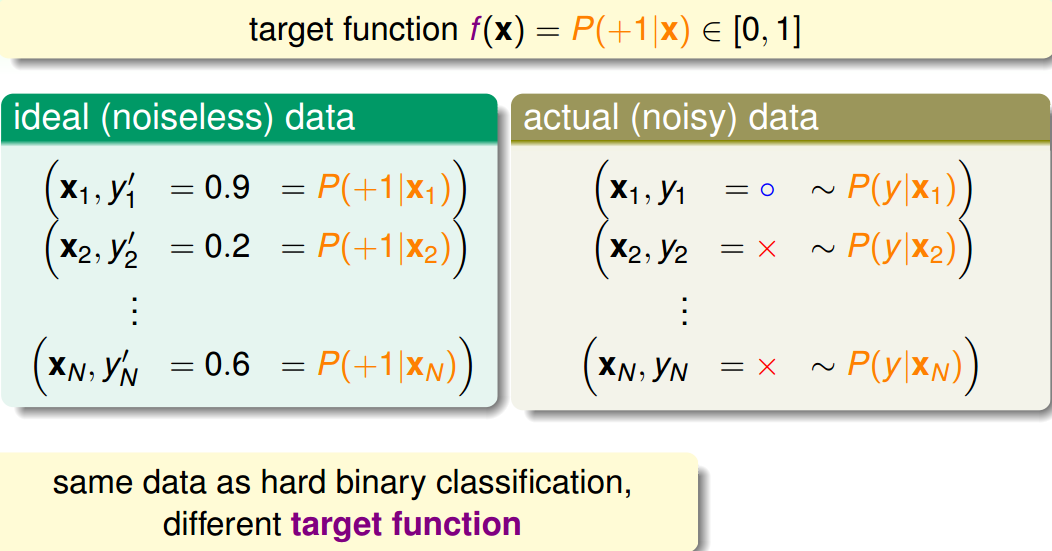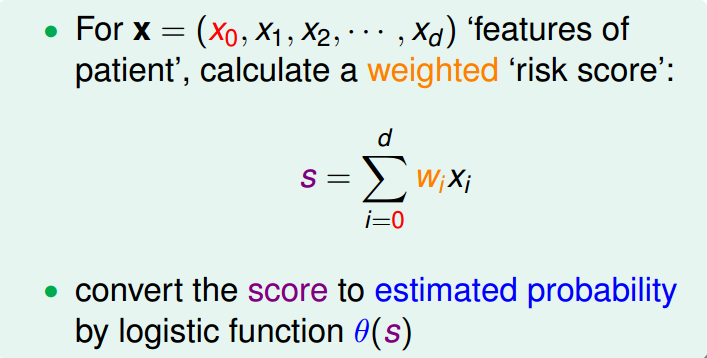h(x)=\theta(w^Tx)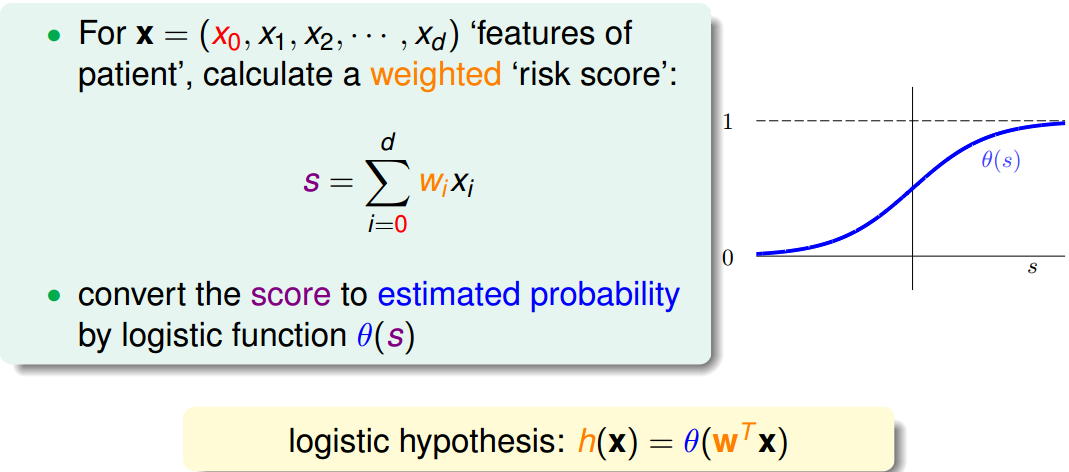## 二、Logistic Regression Error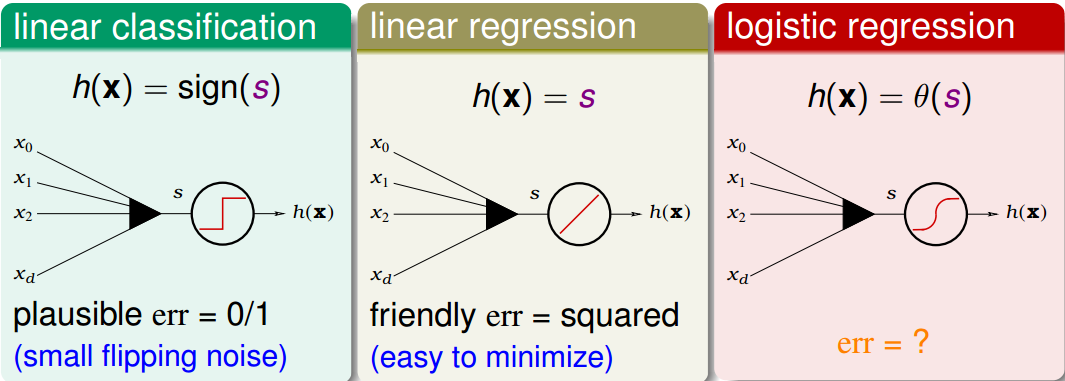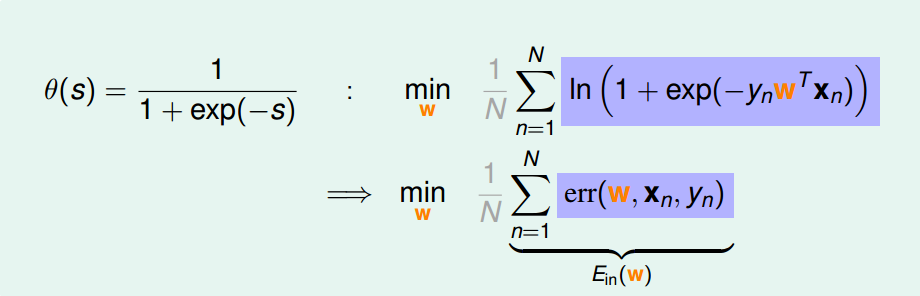## 三、Gradient of Logistic Regression ErrorLogistic Regression的E_{in}是连续、可微、二次可微的凸曲线（开口向上），根据之前Linear Regression的思路，我们只要计算E_{in}的梯度为零时的w，即为最优解。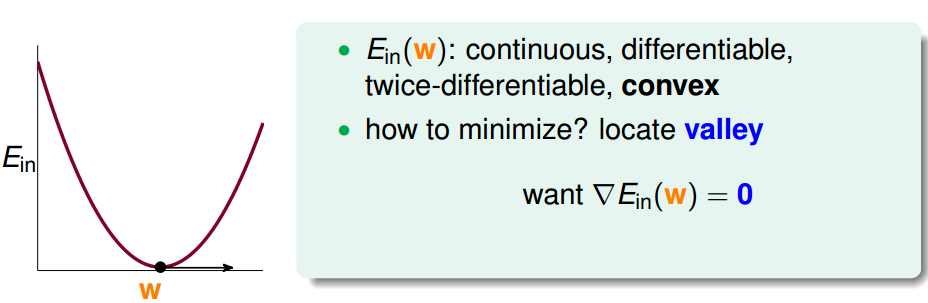E_{in}计算梯度，学过微积分的都应该很容易计算出来：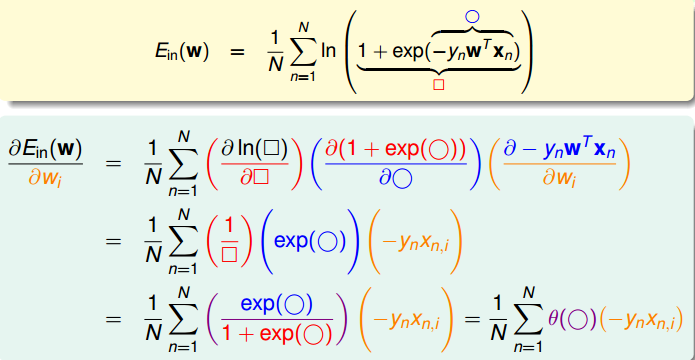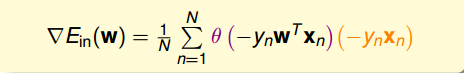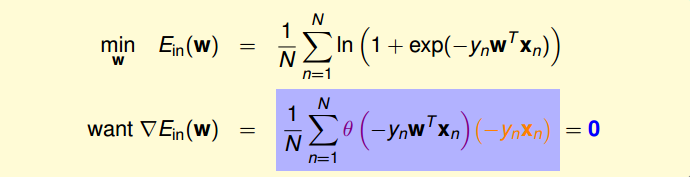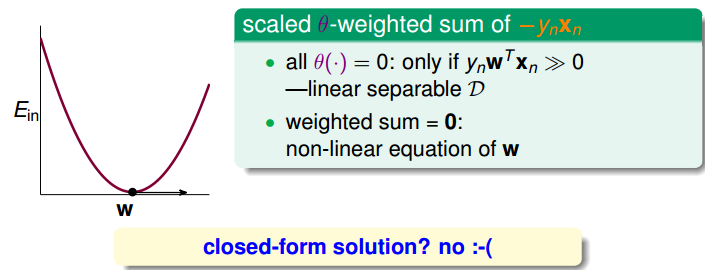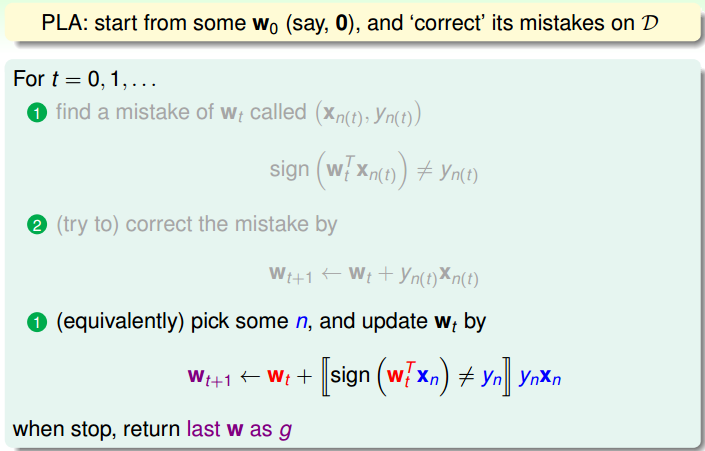w每次更新包含两个内容：一个是每次更新的方向y_nx_n，用v表示，另一个是每次更新的步长\eta。参数(v,\eta)和终止条件决定了我们的迭代优化算法。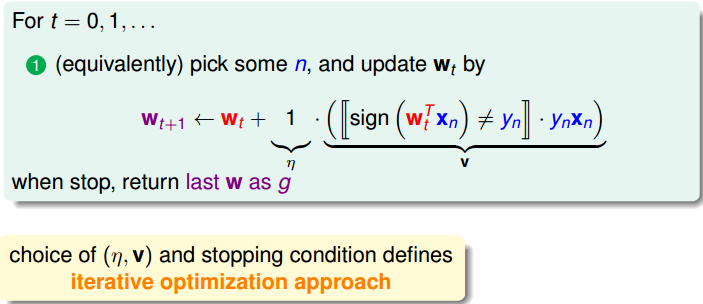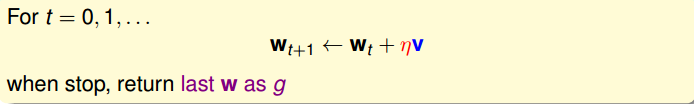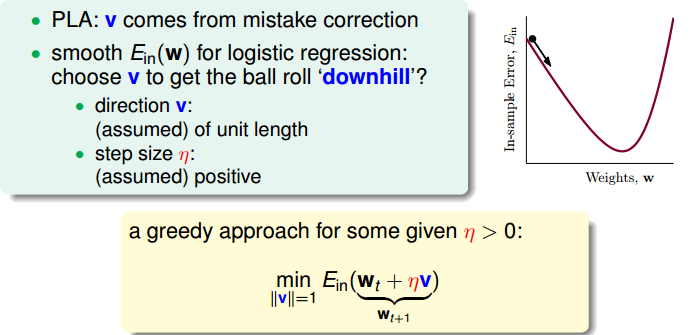E_{in}(w_t+\eta v)\approx E_{in}(w_t)+\eta v^T\nabla E_{in}(w_t)

v=-\frac{\nabla E_{in}(w_t)}{||\nabla E_{in}(w_t)||}

w_{t+1}\leftarrow w_t-\eta\frac{\nabla E_{in}(w_t)}{||\nabla E_{in}(w_t)||}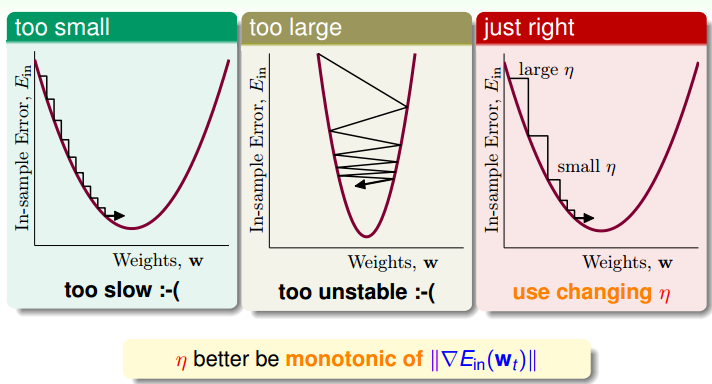w_{t+1}\leftarrow w_t-\eta’\nabla E_{in}(w_t)

\eta’=\frac{\eta}{||\nabla E_{in}(w_t)||}

• 初始化w_0

• 计算梯度\nabla E_{in}(w_t)=\frac1N\sum_{n=1}^N\theta(-y_nw_t^Tx_n)(-y_nx_n)

• 迭代跟新w_{t+1}\leftarrow w_t-\eta\nabla E_{in}(w_t)

• 满足\nabla E_{in}(w_{t+1})\approx0或者达到迭代次数，迭代结束

## 五、总结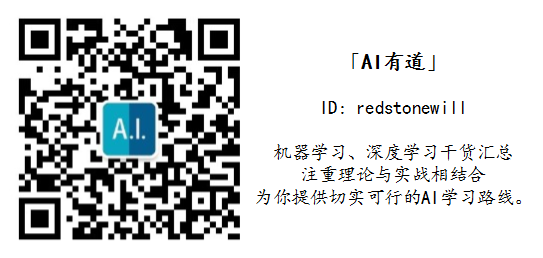### 觉得文章有用就打赏一下文章作者

#### 支付宝扫一扫打赏#### 微信扫一扫打赏